×#### Thank you for registering.

One of our academic counsellors will contact you within 1 working day.

Click to Chat

1800-1023-196

+91-120-4616500

CART 0

• 0

MY CART (5)

Use Coupon: CART20 and get 20% off on all online Study Material

ITEM
DETAILS
MRP
DISCOUNT
FINAL PRICE
Total Price: Rs.

There are no items in this cart.
Continue Shopping```Raoult’s Law

Vapour PressureConsider a pure liquid in a beaker is covered with a jar. Some molecules on the liquid surface evaporate and fill the vacant space available to them. The molecules in the vapour phase move randomly in the vacant space, during this motion, they strike the surface of the liquid  and condensed. This process of evaporation and condensation go on and an equilibrium is established between evaporation and condensation. The pressure exerted by vapours over the liquid surface at equilibrium is called vapour pressure of the liquid.

If solute is non volatile solid or liquid the vapour pressure of solution is equal to partial vapour pressure of solvent in the solution and if the solute is volatile solid or liquid, then vapour pressure will be equal to the sum of partial vapour pressure of solute and that of solvent.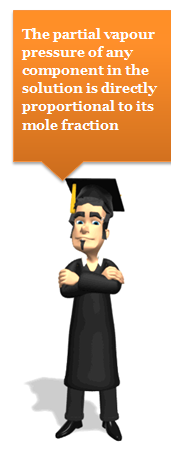Statement of The Roults Law

“The partial vapour pressure of any component in the solution is directly proportional to its mole fraction”. PA = KXA

Where, PA  =  Partial vapour pressure of A

XA  =   Mole fraction of A in solution

For pure liquids XA = 1

Then K = P0A Where P0A  is the vapour pressure of component A in pure state

Hence, PA  = XAP0A

Similarly for component B , PB = XBP0A

Roults law  is applied for a solution of liquid in liquids

Refer to the following video for Raoult’s Law

Raoult’s Law in Combination with Dalton’s Law of Partial Pressure

Assuming that vapours of a liquid is behaving like an ideal gas, then according to Dalton’s law of partial pressure, the total pressure PT is given by

PT = PA + PB

or PT = XAP0A + XBP0B = XAP0A +(1- XA)P0B

Composition of the Vapour:

The composition of the liquid and vapour that are in mutual equilibrium are not necessarily the same, the common sense suggest that the vapour pressure should be richer in the more volatile component. This expectation can be confirmed as follows:

Let the mole fractions of A and B in vapour phase be YA and YB, then from Dalton’s law,Condensation of vapours of solution:

When the vapours of solution (containing liquids A and B) is condensed, the composition of liquids A and B in the condensate remains same. The vapours over condensate can again recondensed and the composition of A and B in condensate (2) remains same as it was in vapour phase over condensate (2).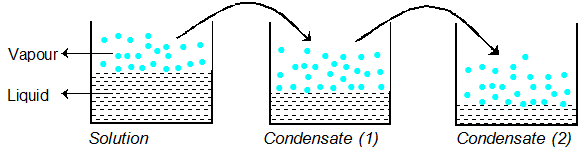Raoult’s Law as a special case of Henry’sLaw

According to Raoult’s law,  pi  = xi pi0. ...(i)

In the solution of a gas in a liquid, one of the components is so volatile that it exists as a gas and we have already seen that its solubility is given by

According to Henry’s law   p = KH x ….......(ii)
If we compare the equations for Raoult’s law and Henry’s law, it can be seen that the partial pressure of the volatile component or gas is directly proportional to its mole fraction in solution. Only the
proportionality constant KH differs from p10.

Thus, Raoult’s law becomes a special case of Henry’s law in which KH becomes equal to p10

Example 1:

Question:

The vapour pressure of two pressure liquids A and B that forms an ideal solution at 300 and 800 torr respectively at temperature T. A mixture of the vapours of A and B for which the mole fraction A is 0.25 is slowly compressed at temperature T. Calculate:

The composition of the first drop of condensation.

The total pressure of this drop formed.

The composition of the solution whose normal boiling point is T.

The pressure when only the last bubble of vapours remains.

Composition of the last bubble.

Solution:

(a)  pB0 = 300 torr pB0 = 800 torr

yA = pA/P = pA0xA/P

where yA and yB are mole fraction of A and B in the vapour

yB = pB0xA/P

∴ yA = yB = pB0xA/pB0xB

0.025/0.75 = 300xA/800xB

i.e.,  xA/xB = 8/9

i.e.,   xA = 8/17 = 0.47

Composition of last drop:

xB = 9/17 = 0.53

(B) Pressure of last drop:

p = pA0xA + pB0xB

(c) At boiling point: p = 760

760o = 300 xA + 800 (1−xA)

xA = 0.08,          ∴ xB = 0.92

(d) For last drop:      xA = 0.25, xB = 0.75

P = 300 × 0.25 + 800 × 0.75 = 675 torr.

(e) yA = pA/P

yA = 300×0.25/675 = 0.111

yB = 0.889

Here, yA and yB are composition of vapour of last drop.

Partial pressure of immiscible liquids

Let ‘A’ and ‘B’ be the two volatile and immiscible liquids; pA and pB be the partial pressures of ‘A’ and ‘B’ respectively.

Then

where mA and mB are molar concentrations of ‘A’ and ‘B’ respectively.

Where WA, WB are weights of ‘A’ and ‘B’ and MA, MB are molecular weights of ‘A’ and ‘B’ respectively.

Ideal and Non – Ideal Solutions

The solutions which obey Raoult’s law at all compositions of solute in solvent at all temperature are called ideal solutions.

Ideal solutions can be obtained by mixing two components with identical molecular size, structure and they should have almost same inter molecular attraction e.g., two liquids A and B form an ideal solution when A –A  and B–B molecular attractions will be same and hence A–B molecular atrraction will be almost same as A–A and B–B molecular attraction.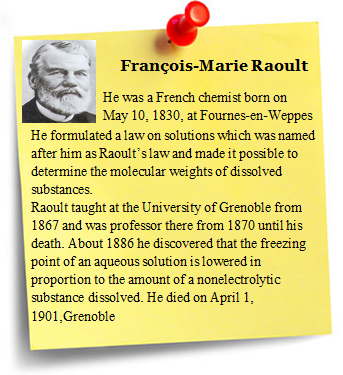An ideal solution should have following characteristics

It should obey Raoult’s law i.e., PA = XA and PB = XB

Hmixing = 0, i.e. no heat should be absorbed or evolved during mixing

Vmixing  = 0, i.e. no expansion or contraction on mixing ?

Examples of  Ideal solutions

Ethyl chloride and ethyl bromide

n–hexane and n–heptane

CCl4 and SiCl4

The solution which deviate from ideal behaviour are called  non ideal solution  or real solutions and they do not obey Raoult’s law over entire range of composition.

It has been found that on increasing dilution, a non ideal solution tend to be ideal.

For non ideal solutions,

PA  XAP0A, PB ¹ XBP0B i.e, they do not obey Raoult’s law

Hmixing  0,

Vmixing  = 0

Here we may have two cases

Case 1:Such a solution show positive deviation from Raoult’s Law and the observed boiling point of such solutions is found to be less than the calculated value. e.g. cyclohexane and ethanol. In ethanol the molecules are held together due to hydrogen bonding,  when cyclohexane is added to ethanol the molecules of cyclohexane tend to occupy the space between ethanol molecules due to which some hydrogen bonds break due to which inter molecular attraction between cyclohexane and ethanol is reduced and hence show higher vapour pressure.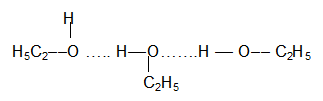Examples of solutions showing positiveedeviation:

Acetone + Ethanol

Acetone- Benzene

Water + Methanol

Water + Ethyanol

Case 2:Solutions of above type show negative deviation from Raoult’s law and their observed boiling point is found to be higher than the calculated value eg.  When acetone and chloroform are mixed together a hydrogen bond is formed between them which increases inter molecular attraction between them and hence decreases the vapour pressure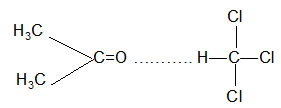Examples of solutions showing negative deviation:

Acetone + Aniline

Chloroform + Diethyl ether

Chloroform + Benzene

Water + HCl

Condition for forming non-ideal solution showing positive deviation

Two liquids A & B on mixing form this type of solutions when

A-B attractive force should be weaker than A-A and B-B attractive force.

“A” and “B” have different shape, size and character.

“A” and “B” escape easily showing higher vapour pressure than the expected value.

Condition for forming non-ideal solution showing negative deviation

Two liquids A & B on mixing form this type of solutions when

A-B attractive force should be greater than A-A and B-B attractive force.

“A” and “B” have different shape, size and character.

Escaping tendency of both “A” and “B” is lower showing lower vapour pressure than expected ideally.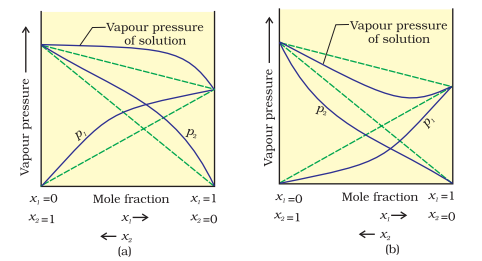Example 2:

Question:

The vapour pressures of ethanol and methanol are 44.5 mm and 88.7 mm Hg respectively. An ideal solution is formed at the same temperature by mixing 60 g of ethanol with 40 g of methanol. Calculate the total vapour pressure for solution and the mole fraction of methanol in the vapour.

Solution:

Mol. mass of ethyl alcohol = C2H2OH = 46

No. of moles of ethyl alcohol = 60/46 = 1.304

Mol. mass of methyl alcohol = CH3OH = 32

No. of moles of methyl alcohol = 40/32 = 1.25

‘XA’, mole fraction of ethyl alcohol = 1.304/1.304+1.25 = 0.5107

‘XB’, mole fraction of methyl alcohol = 1.25/1.304+1.25

Partial pressure of ethyl alcohol = XA. pA0 = 0.5107 × 44.5  = 22.73 mm Hg

Partial pressure of methyl alcohol = XB.pA0 =0.4893 × 88.7 = 43.73 m Hg

Total vapour pressure of solution = 22.73 + 43.40  = 66.13 mm Hg

Mole fraction of methyl alcohol in the vapour  =  Partial pressure of CH3OH/Total vapour pressure = 43.40/66.13 = 0.6563

Azeotropes

The non-ideal solutions showing large deviations from Roult’s law cannot be purified by distillation.

A solution at certain composition which continues to boil at constant temperature without change in the composition of the solution is called an Azeotrope or Constant boiling mixture.

Azeotropes with minimum vapour pressure and Minimum boiling point:

When liquid in a solution do not have great chemical affinity for each other their higher escape tendencies increase the vapour pressure much more than expected on the basis of Roult’s Law. In many cases the deviation are so extreme as to lead to a maximum in the curve. The point of maximum vapour pressure means that the boiling point at this composition will be minimum and constant.

Azeotropes with minimum vapour pressure and Maximum  boiling point:

When liquids in solution show –ve deviation from ideality, their escape tendencies and hence the vapour pressure decreases than expected on the basis of Roult’s Law. In many cases, the maximum in the temperature composition curve is obtained. The point of minimum vapour pressure in the curve means that the boiling point of this composition will be maximum and constant.

Comparison Between Ideal and Non-ideal Solutions

Ideal solutions

Non-ideal solutions

Positive deviation from Raoult’s law

Negative deviation from Raoult’s law

Obey Raoult’s law at every range of concentration.

Do not obey Raoult’s law.

Do not obey Raoult’s law

Hmix = 0; neither is evolved nor absorbed during dissolution.

Hmix>0. Endothermic dissolution; heat is absorbed.

Hmix<0. Exothermic dissolution; heat is evolved.

Vmix = 0; total volume of solution is equal to sum of volumes of the components.

Vmix > 0. Volume is increased after dissolution.

Vmix <0. Volume is decreased during dissolution.

P = pA + pB = pA0XA + pB0XB

pA > pA0XA; pB > pB0XB

∴ pA + pB > pA0XA + pB0XB

pA < pA0XA; pB < pB0XB

∴ pA + pB < pA0XA + pB0XB

A—A, A—B, B—B interactions should be same, i.e., ‘A’ and ‘B’ are identical in shape, size and character.

A—B attractive force should be weaker than A—A and B—B attractive forces. ‘A’ and ‘B’ have different shape, size and character.

A—B attractive force should be greater than A—A and B—B attractive forces. ‘A’ and ‘B’ have different shape, size and character.

Escaping tendency of ‘A’ and ‘B’ should be same in pure liquids and in the solution.

‘A’ and B’ escape easily showing higher vapour pressure than the expected value.

Escaping tendency of both components ‘A’ and ‘B’ is lowered showing lower vapour pressure than expected ideally.

Theory of Fractional Distillation

The process of separation of one liquid from another liquid (binary mixture) having different boiling points by distillation is termed fractional distillation. The information, whether a particular solutions of two liquids can be separated by distillation o not, is provided by the study of liquid-vapour equilibrium diagrams at constant pressure, say atmospheric pressure. The separation is possible only when the vapour phase has a composition different from that of the boiling liquid mixture.

Liquid pairs of type-I (Ideal solutions): The boiling temperature composition curves for liquid and vapour phases in the case of binary solutions of first type are represented in Fig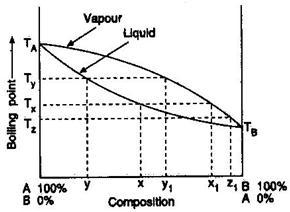Suppose a solution of composition x is heated. When the temperature Tx is reached, the boiling will start. At this temperature the vapour coming off from x will have the composition x1. Since x1 is richer in B than, x, the composition of the residual liquid will become richer will become richer in A. Let the new composition be y. This liquid cannot boil at temperature Tx but will require higher temperature Ty. The vapour coming off at temperature Ty will also be richer in B as represented by y1. Hence the composition of the residue will again be enriched in A. Thus, if the process is allowed to continue, the boiling point of pure liquid A. At the same time the solution becomes more and more richer in A. If the process is continued for a sufficiently long time, pure liquid A can be obtained.

Now, if the initial vapours are condensed, the solution obtained will have the composition x1 and boils at temperature Tz. Evidently, the distillate is richer in B than before. If the process of condensation and redistilling is continued, the final distillate will be pure B component.

Thus, the two liquids forming a solution of Type I can be separated by fractional distillation.

Solution of type-II (Positive deviations from Raoult’s law): The boiling temperature-composition curves of the liquid and vapour phases have been shown in Fig. 5.3. The two curves meet at a minimum point C where the liquid and vapour phases have the same composition. The liquid mixture at point C will boil at constant temperature T without undergoing any change in composition. Such a mixture which boils at constant temperature and distils over completely at the same temperature without any change in composition, is called constant boiling mixture or azeotropic mixture.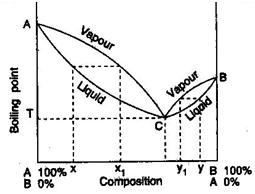Consider the distillation of a mixture of composition x. The vapour given off has the composition x1. The composition of residual liquid will shift towards A. In the mean time the composition of the distillate shifts towards C. ultimately by repeated fractional distillation, the mixture of composition C will be obtained as distillate and pure liquid A will be left as residue. It will never be possible to have pure B.

When a mixture of composition y is distilled, the vapour given off has the composition y1, i.e., the composition of residual liquid will shift towards B. Ultimately the mixture of composition C will be obtained as distillate and pure liquid B will be left as residue. It will never be possible to have pure A.

There are several quid pairs which form minimum boiling point azeotropes. Some examples are given in the following table.

Table: Some azeotropic mixtures

Mixture

% Composition of azeotrope

Boiling point

(pressure = 1 atm)

1.

2.

3.

4.

Water-Ethanol

Pyridine-Water

Ethanol-Benzene

Acetic acid-Toluene

95.97 Ethanol

57.00 Pyridine

32.40 Ethanol

28.00 Acetic-acid

78.13oC

92.6oC

67.8oC

105.4oC

Solutions of type-III (Negative deviations from Raoult’s law): The boiling temperature-composition curves for theliquid and vapour phases have been shown in Fig. 5.4. The curves meet at point C. At this point both liquid and vapour phases have same composition. The constant-boiling mixture has maximum boiling point.

Consider distillation of a mixture of composition x. The vapour coming off is richer in A as indicated by composition x1. The composition of the residual liquid shifts towards C. As the distillation proceeds, the composition of the distillate moves towards A and that of residue towards. C.

Similarly, a mixture of composition lying between B and C, say y, on distillation will gie vapour richer in B as indicated by composition y1. The composition of residual liquid shifts towards C and distillate towards A on repeated distillation.

It is never possible to separate a mixture completely into the pure components A and B. It mainly gives a constant boiling mixture (azeotropes) which can never be separated by distillation.

There are several liquid pairs which form maximum boiling point azeotrope. Some examples are tabulated below:

Mixture

% composition of azeotrope

Boiling point (pressure = 1 atm)

1.

Nitric acid-Water

68% Nitric acid

125.5°C

2.

Acetic acid-Pyridine

65% Pyridine

139.0° C

3.

Chloroform-Aceton

80% Chloroform

65.0° C

4.

Hydrogen chloride-Water

79.8% Water

108.6° C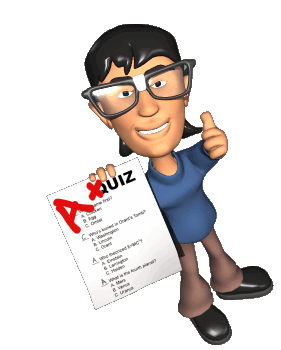Question 1: Enthalpy of mixing  for an  ideal solution is

a. 0

b. -ve

c. +ve

d. may be positive or negative depending on the nature of liquids

Question 2: For pure liquids XA =

a. 0

b. 1

c. 0.1

d. 0.5

Question 3: Which of the followings is not an ideal solution?

a. Ethyl chloride and ethyl bromide

b. n–hexane and n–heptane

c. CCl4 and SiCl4

d. Acetone and Aniline

Question 4: Which of the following solutions does not  show positive deviation?

Acetone  and  Ethanol

Toluene and Benzene

Water and  Methanol

Water and EthyanolQ.1

Q.2

Q.3

Q.4

a

b

d

b

Related Resources

Look here for reference books of chemistry

You can also refer methods of expressing concentration of solution

To read more, Buy study materials of Solutions comprising study notes, revision notes, video lectures, previous year solved questions etc. Also browse for more study materials on Chemistry here.
```### Course Features

• 731 Video Lectures
• Revision Notes
• Previous Year Papers
• Mind Map
• Study Planner
• NCERT Solutions
• Discussion Forum
• Test paper with Video Solution